# Why Can’t I Remember Game of Thrones Characters? An Analysis in R

A quick exploration of Game of Thrones characters names, and the beginning of an answer to the question “Why on earth can’t I remember them all?”

## First, get the data

For this exploration, we first need to get the names of all characters from the show. Quick and easy thanks to the beta serie API.

``````library(httr)
library(jsonlite)
library(tidyverse)
library(magrittr)
api_key <- "XXXX"
``````

Let’s build the API request.

``````# You need id of the serie to request more info

get_beta_serie <- function(show, api_key){
res <- GET(url = paste0("https://api.betaseries.com/shows/search?title=",show),
accept_json(),
"Authorization" = "Bearer c3aa0a5ff128")))
res\$content %>% rawToChar() %>% fromJSON() %>% .\$shows
}

# Get the id

get_beta_id <- function(df, title){
return(df\$id[which(df\$title == title)])
}

GoT_id <- get_beta_serie(show = "game+of+thrones", api_key = api_key) %>%
get_beta_id("Game of Thrones")

# Get characters

get_bet_char <- function(id){
res <- GET(url = paste0("https://api.betaseries.com/shows/characters?id=",id,"&="),
accept_json(),
"Authorization" = "Bearer c3aa0a5ff128")))
res\$content %>% rawToChar() %>% fromJSON() %>% .\$characters
}

GoT_char <- get_bet_char(GoT_id)
nrow(GoT_char)
 98
``````

98 characters… there’s 98 CHARACTERS!

## Something to compare with

### List of series

OK, but before getting really crazy about 98 characters, let’s temperate a little: is this common? To answer that question, we need more DATA.Here, I just have to thank Betaseries again for their cool API :

• which doesn’t limit the number of call you can make
• which can return a random serie from their list
``````# Random serie
get_random_beta <- function(){
res <- GET(url = "https://api.betaseries.com/shows/random?=",
accept_json(),
"Authorization" = "Bearer c3aa0a5ff128")))
res\$content %>% rawToChar() %>% fromJSON() %>% .\$shows %>% .\$id
}
get_random_beta()
 5321

``````

Cool. How about getting mooooooore data.

### Get 1000 random series id, and their characters

``````# 1000 random series
list_id_beta <- replicate(1000, get_random_beta())

# How much unique series?
length(unique(list_id_beta))
 970
list_id_beta %<>% unique

# Get characters for all series
random_char <- map_df(.x = list_id_beta, get_bet_char)

nrow(unique(random_char))
 3811
``````

So, here are 3811 characters from 970 random series picked on the API. Let’s compare!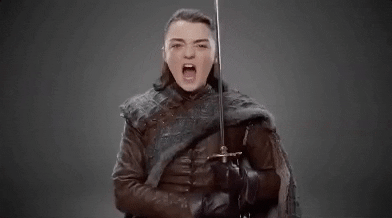### Are there too many characters in Game of Thrones?

``````# Make a full dataframe
random_char\$show_name <- "Not_GoT"
GoT_char\$show_name <- "GoT"
full_char <- rbind(random_char, GoT_char)

# Create a quick theme
custom_theme <- theme_minimal() + theme(plot.title = element_text(hjust = 0.5))

#Visualise
full_char %>%
group_by(show_id) %>%
summarise(char_in_show = n()) %>%
ggplot(aes(x = "", char_in_show)) +
geom_boxplot() +
geom_point(aes(y = 98), color = "red", size = 2) +
coord_flip() +
labs(title = "Number of characters in 970 random series \nand in Game of Thrones (red dot)",
subtitle = " data gathered from BetaSeries API",
x = "",
y = "Number of characters in the show") +
custom_theme
``````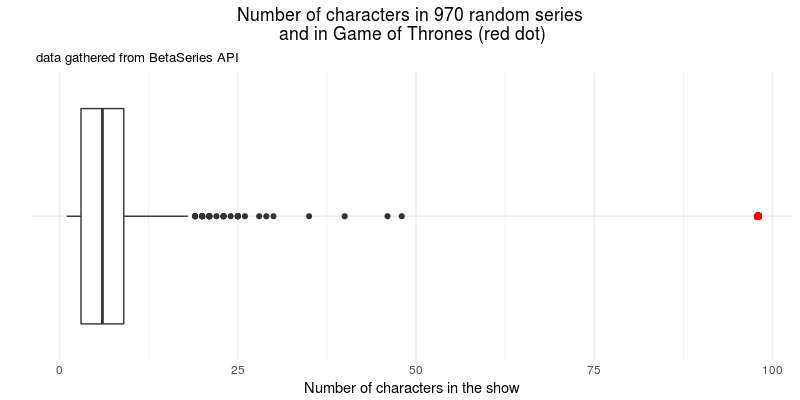OK… So there’s definitely way more characters in Game of Thrones than in other shows — the second most populated show has “only” 48 characters.

But, is there another answer to our original question? (“Why can’t I remember their names?”)

## What’s in a name?

### Length

Let’s formulate some hypotheses. First: maybe their names are too long? I mean, “Melisandre of Asshai” is quite a long name, as is “Xaro Xhoan Daxos”.

``````full_char %>%
group_by(show_id, show_name) %>%
mutate(char_name_length = nchar(name))%>%
ggplot(aes(char_name_length, color = show_name)) +
geom_freqpoly(bins = 50) +
facet_grid(show_name ~., scales = "free_y") +
scale_color_manual(name = "Show", values = c("#973232", "#1E5B5B")) +
labs(title = "Length of characters names in 970 random series and in Game of Thrones",
subtitle = " data gathered from BetaSeries API",
x = "Length of character name in the show",
y = "",
guide = "Show") +
custom_theme
``````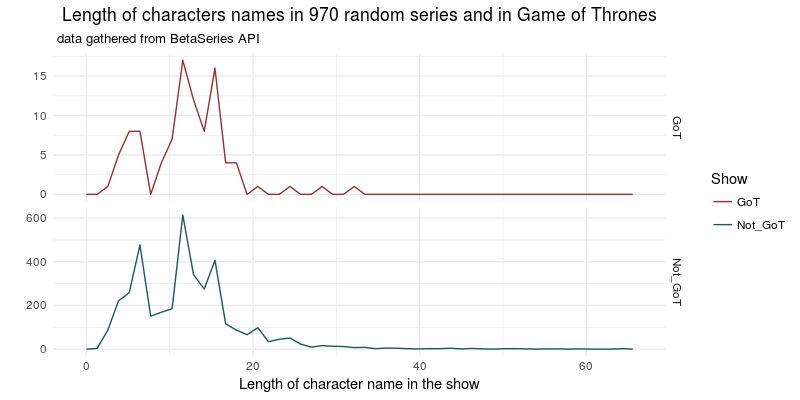Nop, that’s not it either. Let’s try something else.

### Occurences of letters

Are the letters similarly distributed in Game of Thrones and in the other shows?

``````library(stringr)

#Let's clean up a little bit

letter_strip <- function(vec){
vec %>%
tolower() %>%
tm::removePunctuation() %>%
tm::removeNumbers() %>%
str_replace_all(" ", "") %>%
str_replace_all("àäâá", "a") %>%
str_replace_all("éèë", "e") %>%
str_replace_all("ïí", "i") %>%
str_replace_all("ōôó", "o") %>%
str_replace_all("üū", "u") %>%
str_split(pattern = "") %>%
unlist() %>%
iconv(from = "Latin1","ASCII", sub ="") %>%
table() %>%
as.tibble()
}
# That removed 146 non ASCII letters

full_char %>%
group_by(show_id, show_name) %>%
summarise(table_letter = list(letter_strip(name))) %>%
unnest() %>%
rename(letter = ".") %>%
ggplot(aes(letter, n)) +
geom_col() +
facet_grid(show_name ~ ., scale = "free_y") +
labs(title = "Distribution of letters in the name of the characters in 970 random series and in Game of Thrones",
subtitle = " data gathered from BetaSeries API",
x = "",
y = "",
guide = "Show") +
custom_theme

``````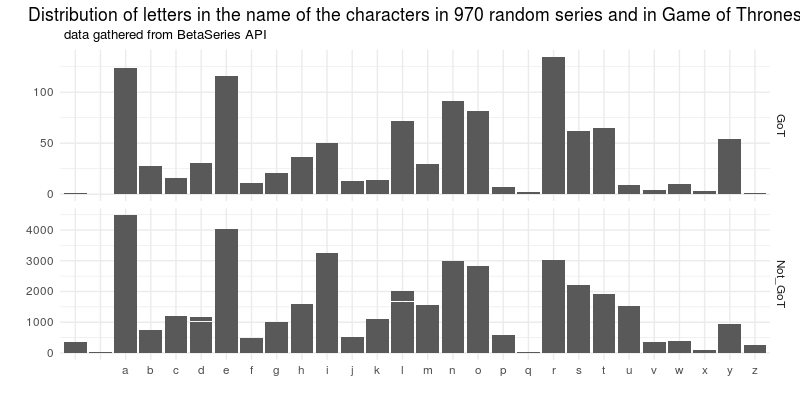Nop, still quite the same. Ok, maybe that’s because their names are composed of repeated letters (remember… Xaro Xhoan Daxos)?

### Repeated letters

``````# Diff letters function

diff_letters <- function(word){
li <- word %>%
tolower() %>%
tm::removePunctuation() %>%
tm::removeNumbers() %>%
str_replace_all(" ", "") %>%
str_split(pattern = "") %>%
unlist
moy <- length(unique(li)) / length(li)
return(moy)
}
# Here, a result of 1 means all letters are different. The closer the result get to zero, the more repeated they are.

diff_letters("Xaro Xhoan Daxos")
 0.5714286
diff_letters("Colin Fay")
 1
``````

Cool. Let’s visualize that.

``````full_char %>%
group_by(id) %>%
mutate(diff_in_letters = diff_letters(name)) %>%
ggplot(aes(diff_in_letters, color = show_name)) +
geom_freqpoly(bins = 50) +
facet_grid(show_name ~., scales = "free_y") +
scale_color_manual(name = "Show", values = c("#973232", "#1E5B5B")) +
labs(title = "Letter difference in the names of characters in 970 random series and in Game of Thrones",
subtitle = " data gathered from BetaSeries API",
x = "Length of character name in the show",
y = "",
guide = "Show") +
custom_theme
``````Ok, nothing fancy here… Now, let’s try some string dist approach.

## String dist

### Chosing a string distance algorithm

My main point while watching GoT is that “every names sound the same”. Like, why on earth should you name your character “Bran” and “Bronn”, “Tyron and Tywinn”, “Tommen” and “Tormund”… and there is literally a “Robb”, a “Robert” and a “Robin”.

Can we see it with string distance? My first intuition is that string distances should be shorter in Game of Thrones than in other shows. Let’s try several methods to find out.

### Levenshtein distance

The Levenshtein distance counts the number of deletions, insertions and substitutions necessary to turn b into a. For example, `stringdist("Colin", "Colis", method = "lv")` returns 1.

``````library(stringdist)

# Just cleaning the names
name_strip <- function(vec){
vec %>%
tolower() %>%
tm::removePunctuation() %>%
str_replace_all(" ", "") %>%
str_replace_all("é", "e") %>%
str_replace_all("à", "a") %>%
str_replace_all("é", "e")
}

string_dist_lv <- function(vec){
stringdistmatrix(vec, method = "lv") %>% as.vector()
}

full_char %>%
mutate(name = name_strip(name)) %>%
group_by(show_id, show_name) %>%
summarize(dist_name_length = list(string_dist_lv(vec = name))) %>%
unnest() %>%
ggplot(aes("",dist_name_length)) +
geom_boxplot() +
coord_flip() +
facet_grid(show_name ~ ., scale = "free_y") +
labs(title = "String distance between the names of the characters \nin Game of Thrones and in 970 random series",
subtitle = "computed using the Levenshtein distance") +
custom_theme
``````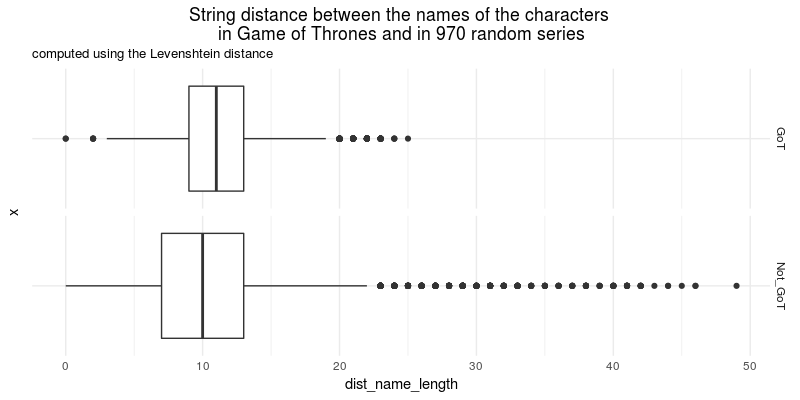OK, Levenshtein is not on my side.

### Longest common substring

This method finds the longest string that can be obtained by pairing characters from a and b, by removing non common characters while keeping the order of characters intact. The result is the number of deletions made to obtain this string.

For example, `stringdist("Colin", "Colis", method = "lcs")` returns 2, because you need to remove the n and the s — the longest common substring being “Coli”. `stringdist("Brann", "Bron", method = "lcs")` returns 3, as you have to remove a, n and n. The lcs is “Brn”. The closer the result is to 0, the closer the strings are.

``````string_dist_lcs <- function(vec){
stringdistmatrix(vec, method = "lcs") %>% as.vector()
}

full_char %>%
mutate(name = name_strip(name)) %>%
group_by(show_id, show_name) %>%
summarize(dist_name_length = list(string_dist_lcs(vec = name))) %>%
unnest() %>%
ggplot(aes("",dist_name_length)) +
geom_boxplot() +
coord_flip() +
facet_grid(show_name ~ ., scale = "free_y") +
labs(title = "String distance between the names of the characters \nin Game of Thrones (red line) and in 970 random series",
subtitle = "computed using the longest common substring method") +
custom_theme
``````Neither on my side…

### Jaro distance

Jaro distance returns a number between 0 and 1, 0 being exact match, 1 complete dissimilarity. `stringdist("Colin", "Colis", method='jw', p=0)` returns 0.1333333. `stringdist("Brann", "Bron", method='jw', p=0)` returns 0.2166667. Let’s try this last one.

``````string_dist_jac <- function(vec){
stringdistmatrix(vec, method='jw', p=0) %>% as.vector()
}

full_char %>%
mutate(name = name_strip(name)) %>%
group_by(show_id, show_name) %>%
summarize(dist_name_length = list(string_dist_jac(vec = name))) %>%
unnest() %>%
ggplot(aes("",dist_name_length)) +
geom_boxplot() +
coord_flip() +
facet_grid(show_name ~ ., scale = "free_y") +
labs(title = "String distance between the names of the characters \nin Game of Thrones and in 970 random series",
subtitle = "computed using the Jaro distance") +
custom_theme
``````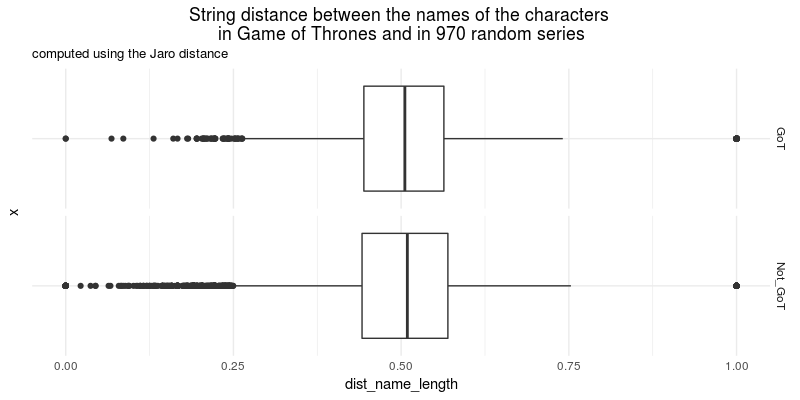Well, Jaro is neither corroborating my initial idea… So, I’ll stick with my original though: there are sooooooo many characters in this show :)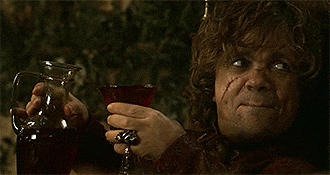Tags:

Categories:

Updated: